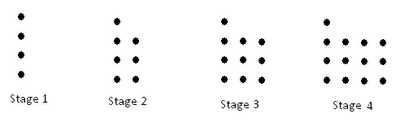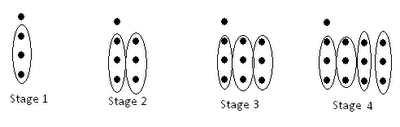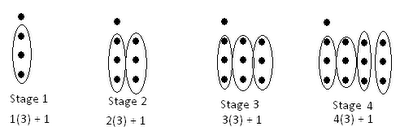## Monday, September 5, 2011

### A different approach to linear equations?

I've been experimenting with something and I think it might be working! So, here it is. I am going to describe briefly how I approach linear reasoning with my 7th-graders and then open it up for feedback.

1. I draw a visual pattern with dots.2. I guide the kids to look for how many dots are added at each stage.

3. The kids visually group dots into n groups of k, where n matches the stage # and k matches the stepwise increase. They'll notice that at each stage, there is a certain number of "leftover" dots.4. Kids start to generate expressions like 1(3) + 1 to represent "1 group of 3, plus 1 extra dot." Then 2(3) + 1 for the next stage, then 3(3) + 1 for the next stage...5. Eventually, kids can figure out that for stage 1000, the number of dots involved would be 1000(3) + 1. (This inductive thinking, it turns out, takes a couple of days to develop and for them to feel comfortable with...)

6. You then coax them into figuring out that for any stage n, the formula is n(3) + 1. It helps to keep pointing in turn at the formula and back at the concrete numerical examples 1(3) + 1, 2(3) + 1, etc. to emphasize that n is just a placeholder.

7. When kids start to gain confidence in their formula, ask them to start making predictions for small stages n=5 or n=6 and then verify their results by actually continuing the visual patterns.

8. After doing this a bunch of times, they then try to do this with numbers instead of visual dots.

The goal is that they should be able to look at a rough "table" that looks like this (with no actual dots drawn):
Stage 1: 16 dots
Stage 2: 20 dots
Stage 3: 24 dots

...and figure out that the algebraic expression for predicting the size at stage n is n(4) + 12, and then also understand that that formula matches 1(4) + 12, 2(4) + 12, 3(4) + 12, etc... One advantage I have already noticed about this method of teaching linear formulas is that it continuously reinforces the idea that the formula is merely an abstraction (or generalization) of an already-existing pattern.

9. Then, when they're comfortable with the entire process, repeat with negative slopes. Instead of circling groups of added dots, they start circling spaces of taken away dots.A kid should be able to look at this (after a bit of guidance) and write the equation y = 16 - x(2) for dots at stage x.

What do you think? Do you think this is another rote method of linear patterns, or do you think this could actually increase student understanding of linear equations? Where do you think I should go from here, to transition their understanding into a formal knowledge of linear patterns within the coordinate plane?

PS. You are welcome to download all my lessons thus far (and planned ahead a bit, even!) here. Teaching 5 grades at once is super fun!!

1.At the stage where they are still gathering, it can be a good idea to write it out more literally: 1 + 3 + 3 + 3, then 1 + 3 + 3 + 3 + 3, then... Eventually a student will point out pulling the 3s together. You can later use the same concept when combining like terms on 1 + x + x + x.

I'd also encourage starting with a "Stage 0", even if it seems clunky at first, since it links nicely to graphs and equation forms. For example, the "16" that magically appears in your #9 wouldn't be magical if you had a Stage 0, and the rule in #8 (12 + 4n) makes a lot more sense if there is a 12 in the table.

(This same issue comes up in sequences and series: sequences that start with "term 1" end up getting messy rules with (n-1) in them, while sequences that start with "term 0" don't. But not in 7th grade!)

Good luck and good work! Seems like the next step is to graph "dots" against "stage". Make sure that when kids write y = 16-2x (or whatever) they can clearly say what x and y stand for. Also bring in some non-examples, like square patterns, to make it clear that not all patterns are linear (a trap kids fall into quickly).

2.I like it! Reminds me of the genre of problems seemingly called "pile patterns", of which you can find many examples thru Google search. Typically the piles are made of little contiguous squares, which leads to an easier (probably) extension to using area formulas. But it's good to see the counting in both ways.

This also reminds me that I need to share my "pile patterns" stuff since my kids (yes, 11th/12th grade) really latched onto this and found that the equations "made sense".

3.@Bowen Thanks for the feedback! I've already done the introductory lessons with the dots with Grade 7, so the 1 + 3 + 3 + 3 ... revisions may have to wait until next year. But, I do like the recommendation of including Stage 0 in the future. I'll do that when we introduce graphing, so they can see the graphical/equation/visual connections.

David Cox also uses something similar, but he uses fractions of a circle as the changing rates. That's also a good next step, once the kids get comfortable graphing/writing equations/making tables for integer coeffs.

4.I love this approach. I agree with brainopennow – the equations now make sense (and they will make sense in different ways as students visualize the figures differently). Not at all rote learning. Without knowing your grade 7 curriculum, I, too, would follow this up with graphing. As an extension, students could try to find the equation of quadratic patterns. Some interesting connections to be made here. See http://wp.me/p1OFpp-3g for more of my thoughts on this approach. Thanks for sharing!